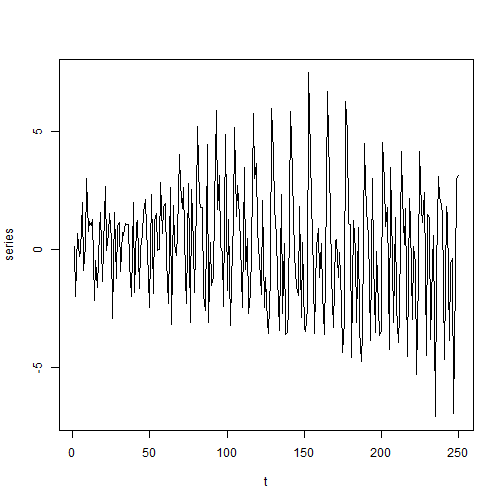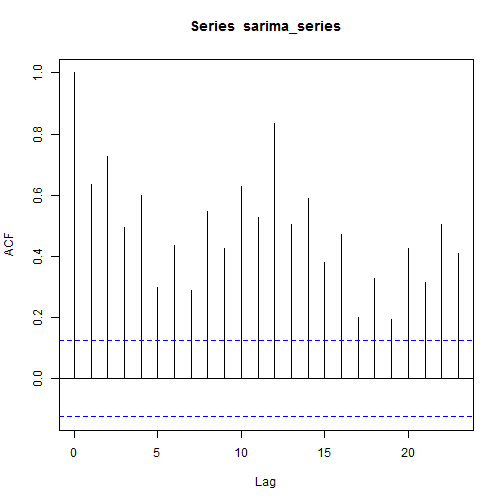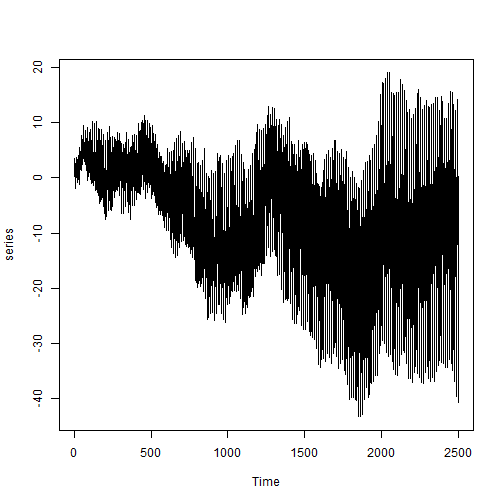# The Case Against Seasonal Unit Roots

There are several ways to model seasonality in a time series. Traditionally, trend-cycle decomposition such as the Holt-Winters procedure has been very popular. Also, until today applied researchers often try to account for seasonality by using seasonal dummy variables. But of course, in a stochastic process it seems unreasonable to assume that seasonal effects are purely deterministic. Therefore, in a time series context seasonal extensions of the classical ARMA model are very popular. One of these extensions is the seasonal unit root model$(1-L^S)X_t=u_t,$

where$LX_t=X_{t-1}$ is the usual lag operator and$S$ is the period length of the seasonality such as$4$ or$12$ for a yearly cycle in quarterly or monthly data and$u_t$ is some short run component such as an$iid$ innovation term or a SARMA(p,q)-(P,Q) model.

I have always been puzzled about the popularity of this process. Probably it is due to the obvious conceptual simplicity. It also seems to be a natural extension of the usual non-seasonal integrated ARIMA model. However, the model generates a feature that we will hardly ever observe in an actual time series: as time progresses the difference between consecutive values of the will become infinitely large.

To see this consider the following example. To generate seasonal unit root processes we first define a function that generates seasonal sums.

seasonal_sum<-function(data,S){
out<-data
for(t in (S+1):length(data)){out[t]<-data[t]+out[(t-S)]}
out
}

We then generate a sample of 250 observations from the process and look at its plot and its autocorrelation function. We choose a period of$S=12$, so that the example resembles a yearly cycle in monthly data.

series<-seasonal_sum(rnorm(250),S=12)
acf(series)ts.plot(series, ylab="series", xlab="t")From the autocorrelation function (ACF) it can be seen that there is a pronounced seasonal behavior with a spike in the ACF at each lag that is an integer multiple of$S$. However, the plot of the series shows a curious behavior. As$t$ increases, we see that the difference between two consecutive observations$\Delta X_t=X_t-X_{t-1}$ increases. This behavior becomes even more pronounced if we increase the sample size to 2500.

ts.plot(seasonal_sum(rnorm(2500),S=12), ylab="series")To understand this feature consider the usual unit root model with an$iid$ innovation$\varepsilon_t$ with variance$\sigma_\varepsilon^2$. This can be expressed as the sum over all past innovations.$X_t=(1-L)^{-1}\varepsilon_t=\sum_{i=0}^t \varepsilon_{t-i}.$

From this representation it is easy to show that the variance of the process is given by$Var(X_t)=t \sigma_\varepsilon^2,$

so that the variance becomes infinite as$t$ approaches infinity. This is a property that seems to apply to many economic and financial time series and is therefore completely reasonable.

Now, the seasonal unit root model can be expressed in a similar way, but with an important twist. To see this, denote the$s$th innovation in the$i$th repetition of the cycle of length$S$ by$\eta_{i}^{(s)}$. This means that if you have monthly observations the innovation in the first January in the sample is$\eta_1^{(1)}$ and the innovation in the second January in the sample is$\eta_2^{(1)}$. By the same principle the innovation in the$4$th December in the sample would be$\eta_4^{(12)}$. Therefore, any observation$X_t=X_{i}^{(s)}$, for some$i=1,..,n$ and$s=1,...,S$ can be represented as$X_i^{(s)}=\sum_{i=1}^n \eta_{i}^{(s)}$.

The important thing to note here is that for two consecutive observations within the$i$th repetition of the cycle we have$X_t=X_i^{(s)}=\sum_{i=1}^n \eta_{i}^{(s)}$ and$X_{t-1}=X_i^{(s-1)}=\sum_{i=1}^n \eta_{i}^{(s-1)}$. Since$\eta_{i}^{(s)}$ and$\eta_{i}^{(s-1)}$ are independent streams of random numbers this means that$X_i^{(s)}$ and$X_i^{(s-1)}$ are independent random walks! Consequently, the difference of the process is given by$\Delta X_t=X_t-X_{t-1}=X_{i}^{(s)}-X_{i}^{(s-1)}=\sum_{i=1}^n \eta_i^{(s)}-\eta_i^{(s-1)},$

so that$Var(\Delta X_t)= 2n Var(\eta_i^{(s)}).$

Since$n$ goes to infinity as$t$ goes to infinity, so does the variance of the changes. Has anybody ever seen a series that exhibits such a feature? Of course in reality we would expect that the innovations are not$iid$ but show some kind of dependence structure, so that the random walks are not completely independent anymore. However, if the dependence is weak – such as that of an ARMA process – they are still asymptotically independent for large lags. Therefore, the same issue arises, as can be seen from the example below.

sarima_sim<-function(T, S, arma_model){
arma_series<-arima.sim(n=T, model=arma_model)
seasonal_sum(data=arma_series, S=S)
}

sarima_series<-sarima_sim(T=250, S=12, arma_model=list(ar=c(0.5,0.3)))

acf(sarima_series)ts.plot(sarima_series, ylab="series")ts.plot(sarima_sim(T=2500, S=12, arma_model=list(ar=c(0.5,0.3))), ylab="series")So what is the conclusion from all this? The seasonal unit root process seems to be ill suited to model most behavior that we observe in practice. However, it is well known that it often generates a good fit. Especially in shorter time series the drawbacks of the seasonal unit root process do not have to become visible. Nevertheless, I think it is fair to say that one could envision a more satisfactory model. One avenue that seems very useful in this context is that of seasonal long memory processes that are able to combine some persistence in the cyclical fluctuations with a finite variance.

Another important conclusion is that we have to be careful with seemingly direct extensions of standard models such as the ARIMA. The fact that the ARIMA is extremely successful in modelling the non-seasonal component, does not necessarily mean that the SARIMA is a good model for the seasonal applications that we have in mind, too.

## 2 thoughts on “The Case Against Seasonal Unit Roots”

1.mark leeds says:

That was a very interesting subtlety and an excellent explanation of it. An example where not inspecting models results carefully ( although with a short series you may not see it anyway ) and using well known techniques blindly can lead to serious problems. Thank you.

Like

2. […] The Case Against Seasonal Unit Roots […]

Like